Courses

# Test: Hydraulic Pump - 2

## 10 Questions MCQ Test Topicwise Question Bank for Mechanical Engineering | Test: Hydraulic Pump - 2

Description
This mock test of Test: Hydraulic Pump - 2 for Mechanical Engineering helps you for every Mechanical Engineering entrance exam. This contains 10 Multiple Choice Questions for Mechanical Engineering Test: Hydraulic Pump - 2 (mcq) to study with solutions a complete question bank. The solved questions answers in this Test: Hydraulic Pump - 2 quiz give you a good mix of easy questions and tough questions. Mechanical Engineering students definitely take this Test: Hydraulic Pump - 2 exercise for a better result in the exam. You can find other Test: Hydraulic Pump - 2 extra questions, long questions & short questions for Mechanical Engineering on EduRev as well by searching above.
QUESTION: 1

### If two pumps identical in all respects and each capable of delivering a discharge Q against a head H are connected in series, the resulting discharge is

Solution: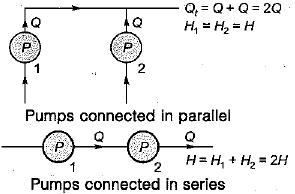QUESTION: 2

Solution:
QUESTION: 3

### In a pump installation the local atmospheric pressure is 10 m of water, vapour pressure head is 0.4 m (abs), height of the pump above sump water level is 5 m. For a head loss in the suction side of 0.6 m, the NPSH is

Solution: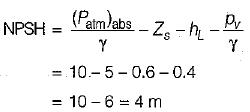Zs = elevation of pump above the liquid surface in the sump. If the pump is set below the liquid level in the sump then Zs would be negative.

QUESTION: 4

Water is to be lifted by a net head of 184 m. Identical pumps with specific speed of 30 and rotative of 1500 rpm capable of discharging 200 L/s are available. The number of pumps required is [Take 1843/4 = 50]

Solution: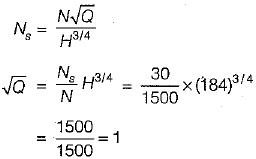∴ Q = 1000 L/s
Number of pumps = 1000/200 = 5

QUESTION: 5

Water is pumped through a pipeline to a hieght of 10 m at the rate of 0.1 m3/s. Frictional and other minor losses are 5 m. Pumping power required, in kW is

Solution:

H = 10 + 5 = 15 m
Pumping power,
P = g Q H = 9:81 x 0.1 x 15
= 14.715 kW

QUESTION: 6

In a reciprocating pump without air vessel, the friction head in the delivery pipe is maximum at the crank angle θ =

Solution: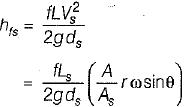hfs is maximum at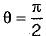QUESTION: 7

The indicator diagram of a reciprocating pump is a plot of

Solution:
QUESTION: 8

Actual indicator diagram for a single stage "reciprocating pump is represented by

Solution:
QUESTION: 9

An air vessel in the delivery side of a reciprocating pump

Solution:

Function ofair vessel fitted on delivery side:
(i) Almost constant delivery discharge is obtained.
(ii) Reduction in friction loss and hence saving in power.

QUESTION: 10

Which one of the following is not a positive displacement pump?

Solution: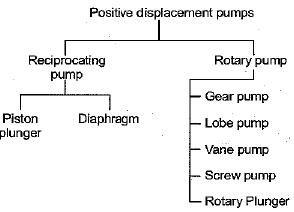Note that centrifugal pump is not positive displacement pump. It is a rotodynamic or dynamic pressure pump.